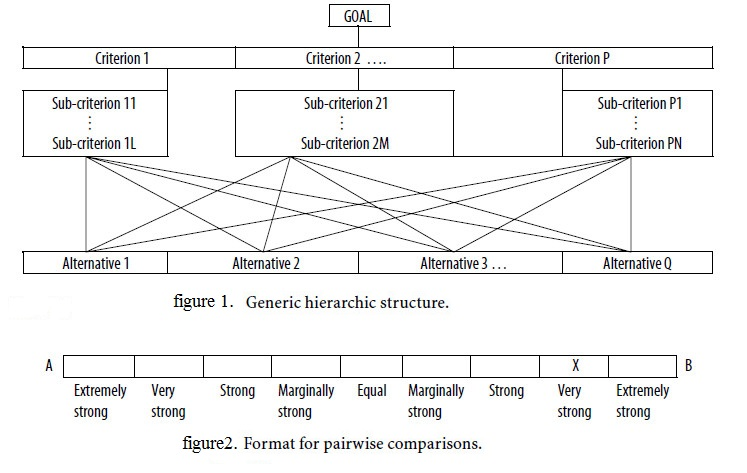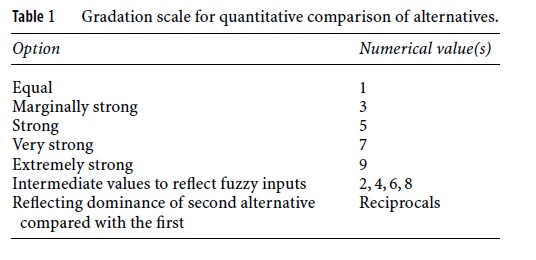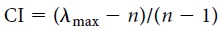Select Page

The AHP provides a means of decomposing the problem into a hierarchy of subproblems which can more easily be comprehended and subjectively evaluated. The
subjective evaluations are converted into numerical values and processed to rank each alternative on a numerical scale. The methodology of the AHP can be explained in following steps:

Step 1: The problem is decomposed into a hierarchy of goal, criteria, sub-criteria and alternatives. This is the most creative and important part of decision-making.
Structuring the decision problem as a hierarchy is fundamental to the process of the AHP. Hierarchy indicates a relationship between elements of one level with those of the level immediately below. This relationship percolates down to the lowest levels of the hierarchy and in this manner every element is connected to every
other one, at least in an indirect manner. A hierarchy is a more orderly form of a network. An inverted tree structure is similar to a hierarchy. Saaty suggests that a
useful way to structure the hierarchy is to work down from the goal as far as one can and then work up from the alternatives until the levels of the two processes are
linked in such a way as to make comparisons possible. Figure 1 shows a generic hierarchic structure.At the root of the hierarchy is the goal or objective of the problem being studied and analysed. The leaf nodes are the alternatives to be compared. In between these two levels are various criteria and sub-criteria. It is important to note that when comparing elements at each level a decision-maker has just to compare with respect to the contribution of the lower-level elements to the upper-level one. This local concentration of the decision-maker on only part of the whole problem is a powerful feature of the AHP.Step 2: Data are collected from experts or decision-makers corresponding to the hierarchic structure, in the pairwise comparison of alternatives on a qualitative
scale as described below. Experts can rate the comparison as equal, marginally strong, strong, very strong, and extremely strong.The opinion can be collected in a
specially designed format as shown in Figure 2.

“X” in the column marked “Very strong” indicates that B is very strong compared with A in terms of the criterion on which the comparison is being made. The comparisons are made for each criterion and converted into quantitative numbers as per Table 1.

Step 3: The pairwise comparisons of various criteria generated at step 2 are organised into a square matrix. The diagonal elements of the matrix are 1. The criterion in the ith row is better than criterion in the jth column if the value of element (i, j) is more than 1; otherwise the criterion in the jth column is better than that in the ith row. The (j, i) element of the matrix is the reciprocal of the (i, j) element.Step 4: The principal eigenvalue and the corresponding normalised right eigenvector of the comparison matrix give the relative importance of the various criteria being compared. The elements of the normalised eigenvector are termed weights with respect to the criteria or sub-criteria and ratings with respect to the  alternatives.

Step 5: The consistency of the matrix of order n is evaluated.Comparisons made by this method are subjective and the AHP tolerates inconsistency through the amount of redundancy in the approach. If this consistency index fails to reach a required level then answers to comparisons may be re-examined. The consistency index, CI, is calculated aswhere max is the maximum eigenvalue of the judgement matrix. This CI can be compared with that of a random matrix, RI. The ratio derived, CI/RI, is termed the consistency ratio, CR. Saaty suggests the value of CR should be less than 0.1.

Step 6: The rating of each alternative is multiplied by the weights of the sub-criteria and aggregated to get local ratings with respect to each criterion. The local ratings are then multiplied by the weights of the criteria and aggregated to get global ratings.

The AHP produces weight values for each alternative based on the judged importance
of one alternative over another with respect to a common criterion.